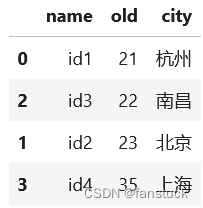# PySpark数据分析基础：PySpark Pandas创建、转换、查询、转置、排序操作详解

1.Series

2.DataFrame

3.Time-Series

4.Panel

5.Panel4D

6.PanelND

1.引入库

2.转换实现

pyspark pandas series创建

pyspark pandas dataframe创建

from_pandas转换

Spark DataFrame转换

三、PySpark Pandas操作

1.读取行列索引

2.内容转换为数组

3.DataFrame统计描述

4.转置

5.排序

按某列值排序

# 前言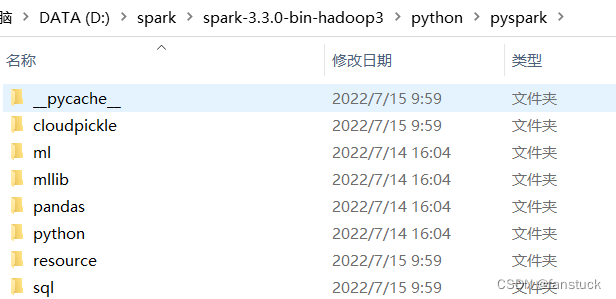PySpark数据分析基础：PySpark基础功能及基础语法详解

# 一、Pandas数据结构

Pandas数据结构是通用了，共有六种数据结构，想要详细了解可以去看我这篇文章：一文速学-数据分析之Pandas数据结构和基本操作代码

## 1.Series

Series要理解很简单，就像它的单词为系列的意思。类似与数据结构中的字典有索引和对应值，也可以理解为数组，在Series中的下标1,2,3,…索引对应它的不同值。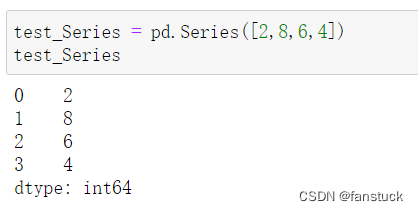## 2.DataFrame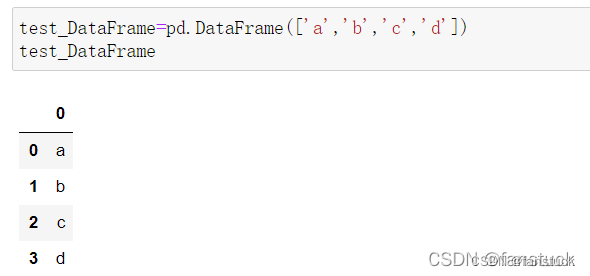## 3.Time-Series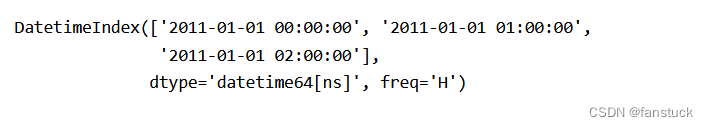## 4.Panel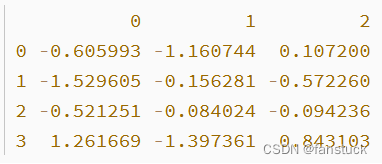# 二、Pyspark实例创建

## 1.引入库

``````import pandas as pd
import numpy as np
import pyspark.pandas as ps
from pyspark.sql import SparkSession

``````

``````import os
os.environ["PYARROW_IGNORE_TIMEZONE"] = "1"
``````

## 2.转换实现

### pyspark pandas series创建

``s = ps.Series([1, 3, 5, np.nan, 6, 8])``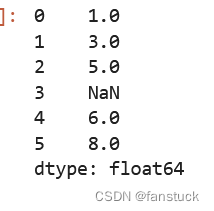### pyspark pandas dataframe创建

``````ps_df=ps.DataFrame(
{'name':['id1','id2','id3','id4'],
'old':[21,23,22,35],
'city':['杭州','北京','南昌','上海']
},
index=[1,2,3,4])``````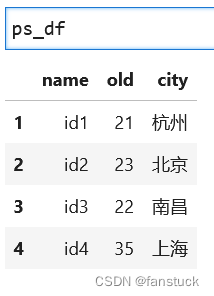### from_pandas转换

``````pd_df=pd.DataFrame(
{'name':['id1','id2','id3','id4'],
'old':[21,23,22,35],
'city':['杭州','北京','南昌','上海']
},
index=[1,2,3,4])``````

``````ps_df=ps.from_pandas(pd_df)
type(ps_df)``````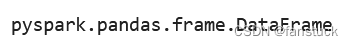### Spark DataFrame转换

``````spark = SparkSession.builder.getOrCreate()
sp_df=spark.createDataFrame(pd_df)
sp_df.show()``````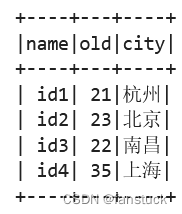``ps_df=sp_df.pandas_api()``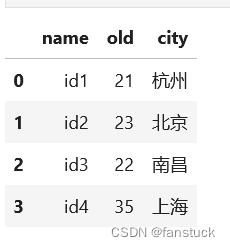且和pandas一样查看数据类型方法是一样的：

``ps_df.dtypes``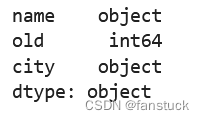Spark DataFrame中的数据在默认情况下并不保持自然顺序

``ps_df.head()``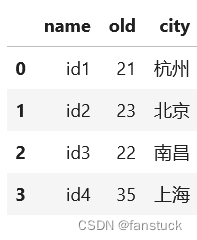# 三、PySpark Pandas操作

## 1.读取行列索引

``ps_df.index``
`Int64Index([0, 1, 2, 3], dtype='int64')`

``ps_df.columns``
`Index(['name', 'old', 'city'], dtype='object')`

## 2.内容转换为数组

``ps_df.to_numpy()``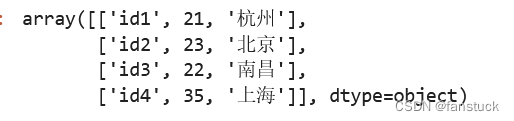## 3.DataFrame统计描述

``ps_df.describe()``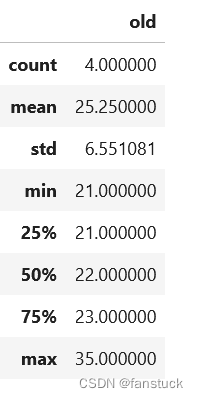## 4.转置

``ps_df.T``

``````ps_df['old']=ps_df['old'].astype(str)
ps_df.T``````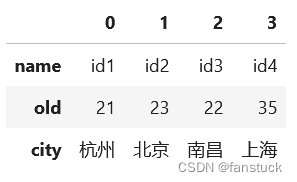## 5.排序

### 按行索引排序

``ps_df.sort_index(ascending=False)``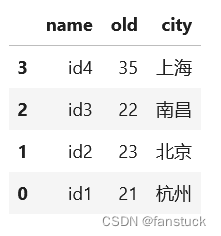### 按某列值排序

``ps_df.sort_values(by='old')``